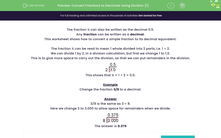# Convert Fractions to Decimals Using Division (1)

In this worksheet, students use division to convert simple fractions into their decimal equivalents.Key stage:  KS 2

Curriculum topic:   Number: Fractions, Decimals and Percentages

Curriculum subtopic:   Calculate Fraction-Decimal Equivalents

Difficulty level:#### Worksheet Overview

The fraction ½ can also be written as the decimal 0.5.

Any fraction can be written as a decimal.

This worksheet shows how to convert a simple fraction to its decimal equivalent.

The fraction ½ can be read to mean 1 whole divided into 2 parts, i.e. 1 ÷ 2.

We can divide 1 by 2, in a division calculation, but first we change 1 to 1.0.

This is to give more space to carry out the division, so that we can put remainders in the division.This shows that ½ = 1 ÷ 2 = 0.5.

Example

Change the fraction 3/8 to a decimal.

3/8 is the same as 3 ÷ 8.

Here we change 3 to 3.000 to allow space for remainders when we divide.